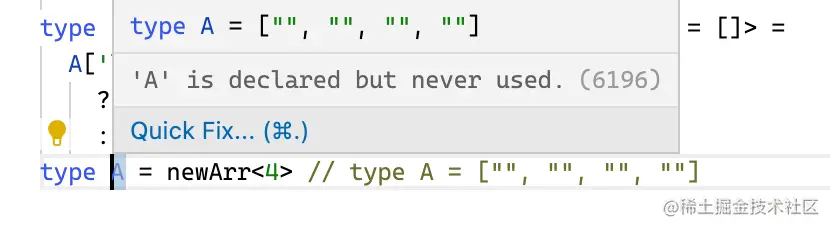# TS 类型体操还能这么玩，太秀了！

12994

• 巧用数组上数学课
• 模版字符串为所欲为
• 中序遍历 TS 也能行
• infer + 递归随意秒杀

## 一、巧用数组上数学课

``````GreaterThan<2, 1> //should be true
GreaterThan<1, 1> //should be false
GreaterThan<10, 100> //should be false
GreaterThan<111, 11> //should be true
``````

• 第一种就是递归，但是通过实践我们会知道，当参数过大时，很容易爆栈
• 第二种方法就是构造两个长度为 `T``U` 的数组，通过数组判断哪个数组更长

### 递归法

• 思路是拿一个新数组，和 `T`,`U` 进行对比，哪个先追上新数组的长度，哪个就小
• 简单一点来说就是，两个不一样长的木棍放在一起，我们从一端开始不断往前走，先摸到的那个木棍就是短一点的

``````// 答案
type GreaterThan<T extends number, U extends number, R extends any[] = []> =
T extends R['length']
? false
: U extends R['length']
? true
: GreaterThan<T, U, [...R, 1]>
``````

### 构造数组

``````[1, 1, 1, 1] extends [1, 1] ? true : false // false
[1, 1] extends [1, 1, 1] ? true : false // false
``````

``````// 伪代码
A extends [...A, ...any] ? true : false
``````

``````type newArr<T extends number, A extends any[] = []> =
A['length'] extends T
? A
: newArr<T, [...A, '']>
``````

``````type A = newArr<4> // type A = ["", "", "", ""]
````````````type GreaterArr<T extends any[], U extends any[]> = U extends [...T, ...any] ? false : true
``````

``````type GreaterThan<T extends number, U extends number> = GreaterArr<newArr<T>, newArr<U>>
``````

## 二、模版字符串为所欲为

``````type ClassNames1 = BEM<'btn', ['price']> // 'btn__price'
type ClassNames2 = BEM<'btn', ['price'], ['warning', 'success']> // 'btn__price--warning' | 'btn__price--success'
type ClassNames3 = BEM<'btn', [], ['small', 'medium', 'large']> // 'btn--small' | 'btn--medium' | 'btn--large'
``````

b 是第二个参数，那么就用 `__` 来连接，c 是第三个参数就用 `--` 来连接

``````// 数组转联合
T[number]
// 对象转联合
Object[keyof T]
``````

``````type BEM<B extends string, E extends string[], M extends string[]> = `\${B}__\${E[number]}--\${M[number]}`
``````

``````type IsNever<T> = [T] extends [never] ? true : false
type IsUnion<U> = IsNever<U> extends true ? "" : U
type BEM<B extends string, E extends string[], M extends string[]> = `\${B}\${IsUnion<`__\${E[number]}`>}\${IsUnion<`--\${M[number]}`>}`
``````

## 三、中序遍历 TS 也能行

JS 实现中序遍历，你闭着眼睛就能写，那 TS 呢

``````const tree1 = {
val: 1,
left: null,
right: {
val: 2,
left: {
val: 3,
left: null,
right: null,
},
right: null,
},
} as const

type A = InorderTraversal<typeof tree1> // [1, 3, 2]
``````

``````const inorderTraversal = (root) => {
if(!root) return []
const res = []
while(root) {
inorderTraversal(root.left)
res.push(val)
inorderTraversal(tree.right)
}
return res
}
``````

JS 是在 root 为 `null` 时结束。对于 `TS` 来说，实现递归，需要 `extends TreeNode` 而不是 `null` 来结束

``````// 答案
interface TreeNode {
val: number
left: TreeNode | null
right: TreeNode | null
}
type InorderTraversal<T extends TreeNode | null> =
[T] extends [TreeNode]
? (
[
...InorderTraversal<T['left']>,
T['val'],
...InorderTraversal<T['right']>
]
)
: []
``````

## 四、infer + 递归随意秒杀

`infer` 可谓是 TS 中的大杀器，大多数题目都会涉及到它的使用，他可以很方便的帮我们推断出一个变量的类型，我们看看这道题

``````type Res = Without<[1, 2], 1> // expected to be 
type Res1 = Without<[1, 2, 4, 1, 5], [1, 2]> // expected to be [4, 5]
type Res2 = Without<[2, 3, 2, 3, 2, 3, 2, 3], [2, 3]> // expected to be []
``````

• 如果能够被 `U` 包含，那就丢弃，也就是把剩余的递归，不保留这一项
• 如果不包含，那就用 `[R, ...]` 把它给留下，剩下的继续递归 因此很有可能写下这样的代码
``````type Without<T, U> =
T extends [infer R, ...infer F]
? R extends U
? Without<F, U>
: [R, ...Without<F, U>]
: T
``````

``````type ToUnion<T> = T extends any[] ? T[number] : T
type B = ToUnion<['1','b']> // type B = "1" | "b"
``````

``````// 答案
type ToUnion<T> = T extends any[] ? T[number] : T
type Without<T, U> =
T extends [infer R, ...infer F]
? R extends ToUnion<U>
? Without<F, U>
: [R, ...Without<F, U>]
: T
``````

## 总结

• 巧用辅助变量
• 遇到计算时大胆使用 `['length']`
• 诡异字符串操作多用模版字符串
• infer + 递归大杀器